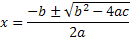A | B | C | D | E | F | G | H | I | J | K | L | M | N | O | P | Q | R | S | T | U | V | W | X | Y | Z

The value x can be calculated from the Quadratic Equation using the formulaHere b2- 4ac is represented using Upper case Letter 'D' called Discriminant.

Here a, b, c are coefficients in the Quadratic Equation   ( ax2 + bx + c ; a ≠ 0 )